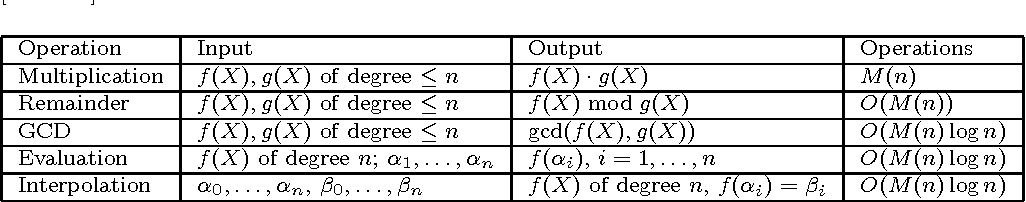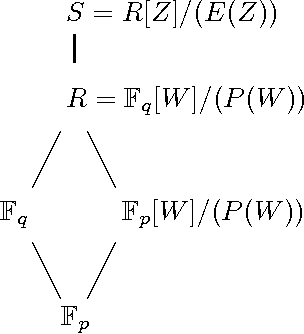You are currently offline. Some features of the site may not work correctly.

# Factorization of polynomials over finite fields

Known as: Factoring polynomials over finite fields, Polynomial factorization over finite fields, Distinct degree factorization
In mathematics and computer algebra the factorization of a polynomial consists of decomposing it into a product of irreducible factors. This… Expand
Wikipedia

## Papers overview

Semantic Scholar uses AI to extract papers important to this topic.
Highly Cited
2011
Highly Cited
2011
• SIAM J. Comput.
• 2011
• Corpus ID: 412751
We obtain randomized algorithms for factoring degree \$n\$ univariate polynomials over \$F_q\$ requiring \$O(n^{1.5 + o(1)} log^{1+o… Expand
••Is this relevant?
2004
2004
• J. Symb. Comput.
• 2004
• Corpus ID: 29836744
A deterministic polynomial time algorithm is presented for finding the distinct-degree factorization of multivariate polynomials… Expand
Is this relevant?
2004
2004
In the following, we describe a way of factoring polynomials in Fq[X] with Drinfeld modules. We furthermore analyse the… Expand
Is this relevant?
1993
1993
Abstract The recently developed algorithm of Niederreiter for the factorization of polynomials over finite fields provides a… Expand
Is this relevant?
1993
1993
• J. Symb. Comput.
• 1993
• Corpus ID: 206574135
Abstract A new factorization algorithm for polynomials over finite fields was recently developed by the first author. For finite… Expand
Is this relevant?
1992
1992
Let p be a prime and F be a polynomial with integer coefficients. Suppose that the discriminant of F is not divisible by p… Expand
Is this relevant?
1991
1991
Abstract It is shown that, assuming the generalized Riemann hypothesis , there exists a deterministic polynomial time algorithm… Expand
Is this relevant?
Highly Cited
1990
Highly Cited
1990
We present a new deterministic algorithm for factoring polynomials over Zp of degree n. We show that the worst-case running time… Expand
Is this relevant?
Highly Cited
1987
Highly Cited
1987
• Lajos Rónyai
• 28th Annual Symposium on Foundations of Computer…
• 1987
• Corpus ID: 16360930
We propose a new deterministic method of factoring polynomials over finite fields. Assuming the Generalized Riemann Hypothesis… Expand
Is this relevant?
Highly Cited
1962
Highly Cited
1962

Is this relevant?